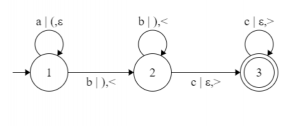# L-graphs and what they represent in TOC

• Difficulty Level : Expert
• Last Updated : 20 Nov, 2019

Prerequisite – Finite automata introduction
All programming languages can be represented as a finite automata. C, Paskal, Haskell, C++, all of them have a specific structure, grammar, that can be represented by a simple graph. Most of the graphs are NFA’s or DFA’s. But NFA’s and DFA’s determine the simplest possible language group: group of regular languages [Chomsky’s hierarchy]. This leaves us with a question: what about all other types of languages? One of the answers is Turing machine, but a Turing machine is hard to visualize. This is why in this article I will tell you about a type of finite automata called an L-graph.

In order to understand how L-graphs work we need to know what type of languages L-graphs determine. To put it simply, L-graphs represent context-sensitive type of languages [and every other type that the context-sensitive group contains]. If you don’t know what “context-sensitive” means, let me show you an example of a language that can be represented by an L-graph and not by any easier type of finite automata.

This language is. Corresponding L-graph looks like this:As you can see the brackets after the symbol ‘|’ control the numbers of symbols that come after the symbols ‘a’. This leads us to the two features that all L-graphs possess: all L-graphs have up to two independent from each other and from input symbols bracket groups, both bracket groups have to be right [string from a Dyck language] in order for the string of input symbols to be accepted by the given L-graph.

You can see that an L-graph is just a version of finite automata with an added couple of bracket groups. To help you get an understanding of why the languages determined by L-graphs are context-sensitive, check what strings the L-graph shown above has to accept.To conclude, I would like to add three other definitions that I’ll be using in the future. These definitions are very important for the hypothesis [and its future proof or disproof]. Refer – Hypothesis (language regularity) and algorithm (L-graph to NFA)

We will call a path in the L-graph neutral, if both bracket strings are right. If a neutral path T can be represented like this, T =, whereandare cycles andis a neutral path (,orcan be empty), T is called a nest. We can also say that the three (,,) is a nest or thatandform a nest in the path T.

(, d)-core in an L-graph G, defined as Core(G,, d), is a set of (, d)-canons. (, d)-canon, whereand d are positive whole numbers, is a path that contains at most m,, neutral cycles and at most k, kd, nests that can be represented this way:is part of the path T,, i = 1 or 3,, are cycles, every pathis a nest, where=,.

The last definition is about a context free L-graph. An L-graph G is called context free if G has only one bracket group (all rules in the L-graph have only one look of these two: [‘symbol’ | ‘bracket’, ?] or [‘symbol’ | ?, ‘bracket’]).

[Definition of a Dyck language.andare disjoint alphabets. There exists a bijection (function that for every element from the 1st set matches one and only one element from the 2nd set). Then the language defined by the grammar,, we will call a Dyck language.]

My Personal Notes arrow_drop_up
Recommended Articles
Page :